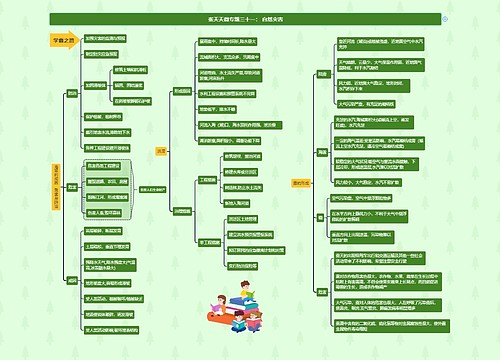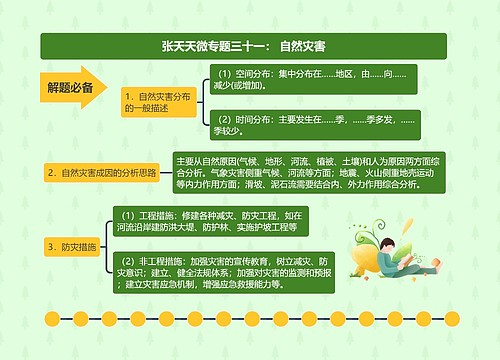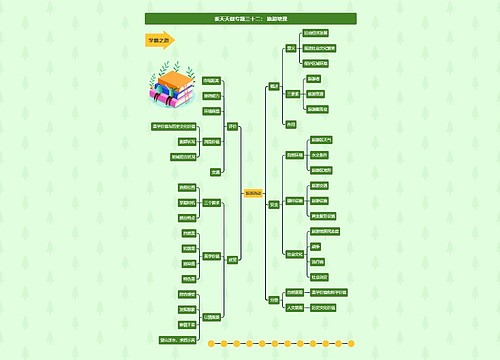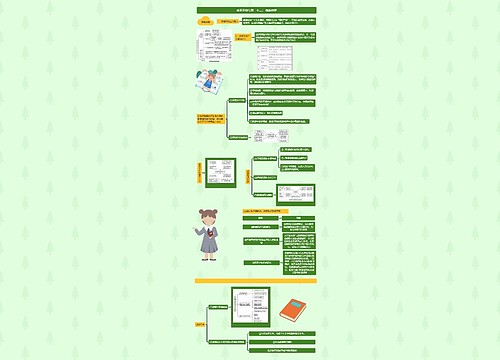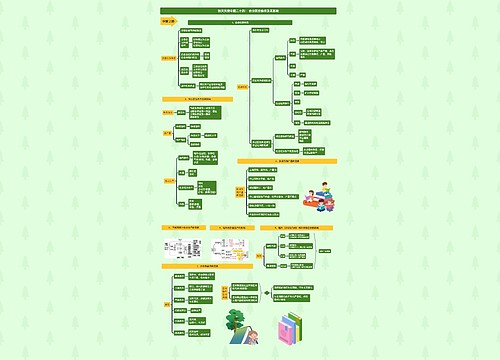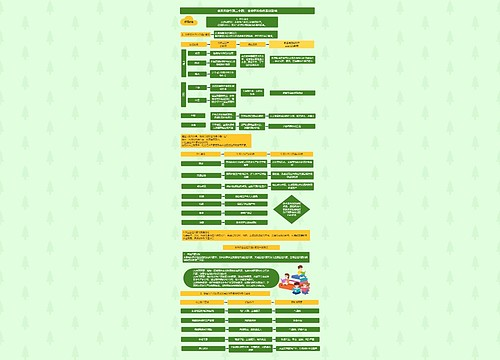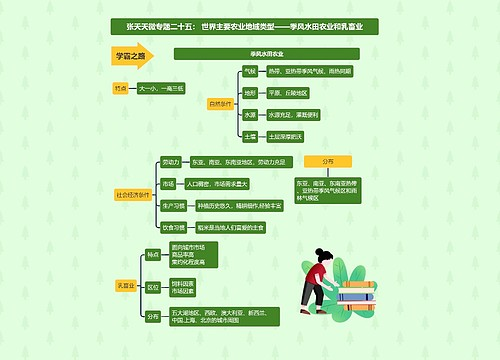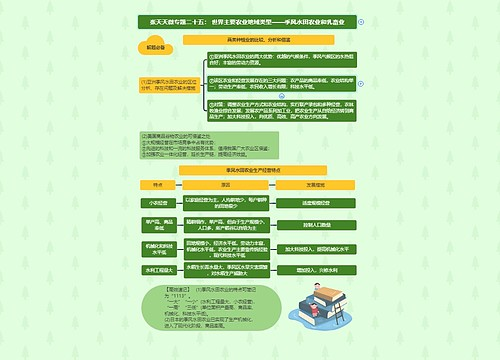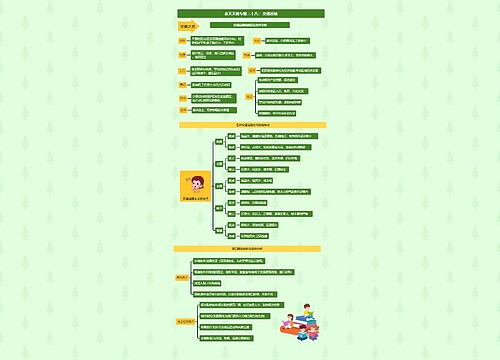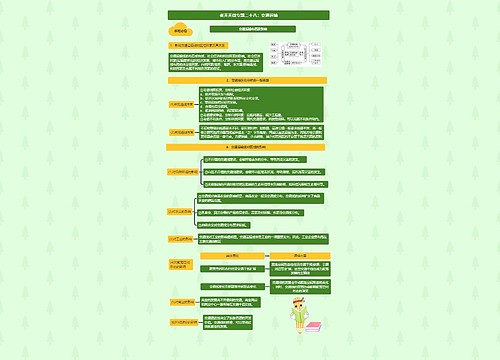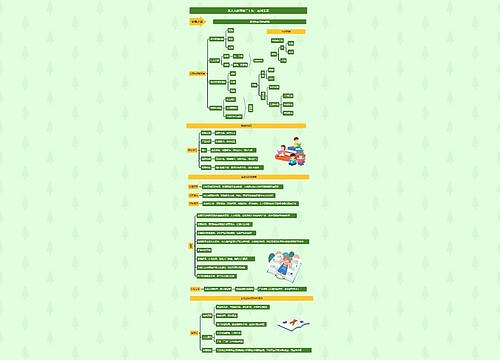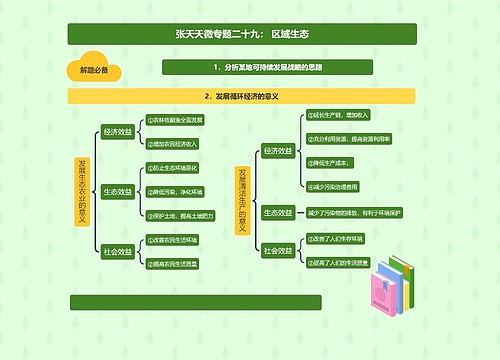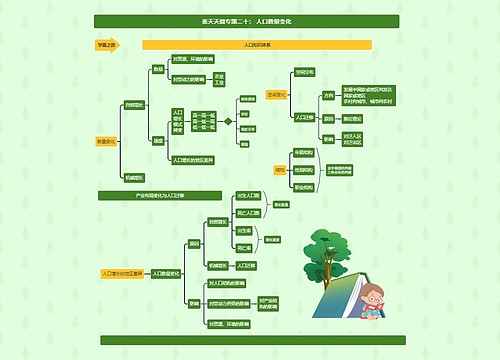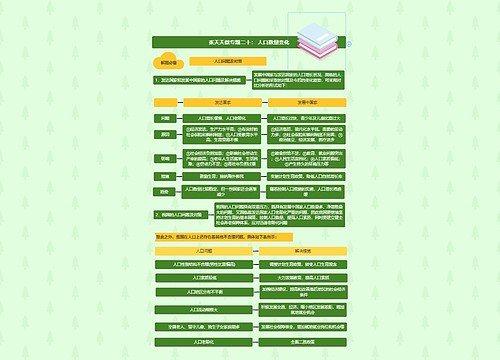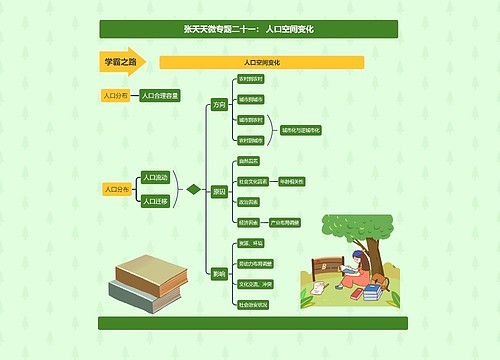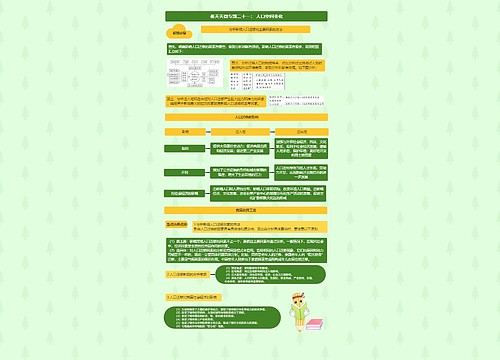AI对话就去对话鸭，GO>>

# ﻿信源与信源熵思维导图

收藏
分享ζั͡ ั͡✾　 浏览量：492023-03-26 15:57:17

﻿信源与信源熵知识点

﻿信源与信息熵思维导图模板大纲

## ﻿信源

﻿所发出的各个符号是相互独立的，发出的符号序列中的各个符号之间没有统计关联性

﻿发出单个符号的无记忆信源

﻿发出符号序列的无记忆信源

﻿有记忆信源

﻿所发出的各个符号的概率是有关联的

﻿离散有记忆信源

﻿发出符号序列的马尔可夫信源

## ﻿自信息量

﻿I(xi）=log1/p(xi)=-log p(xi)

﻿以2为底，单位为比特（bit）

﻿以e为底，单位为奈特（nat）

﻿以10为底，单位为笛特（det）

﻿自信息量：符号出现后，提供给收信者的信息量

﻿联合自信息量

﻿I（xi,yj）=log1/p(xi,yj)=-log p(xi,yj)

﻿I（xi I yj）=log1/p(xi I yj)=-log p(xi I yj)

## 离散信源熵

﻿信源中各个符号自信息量的数学期望

H(X)=E(I(x))=∑p(x)I(x)=-∑p(x)log p(x)

﻿条件熵H(X I Y)

## 互信息

﻿I(X;Y)=H(X)-H(X I Y)

I(X;Y)=H(Y)-H(Y I X)

﻿平均互信息量

﻿对称性

﻿非负性

﻿极值性

﻿凸函数性

﻿单符号离散信源互信息

﻿性质

﻿对称性

﻿相互独立时，互信息为0

﻿互信息量可为正值或负值

﻿条件互信息量

﻿联合互信息量

## ﻿数据处理中信息的变化

﻿数据处理定理：当消息通过多级处理器时，随着处理器数目增多，输入消息与输出消息间的平均互信息量趋于变小

## ﻿相对熵和熵的性质

﻿相对熵

D(p//q)=∑plog p/q

﻿性质

﻿D(p//q)≠D(q//p)

D(p//q)≥0

﻿熵的性质

﻿非负性

﻿确定性

﻿对称性

﻿香农辅助定理(极值性)

﻿最大熵定理

﻿条件熵小于无条件熵

﻿扩展性

﻿递增性

﻿维拉图

﻿离散无记忆信源的序列熵

﻿离散有记忆信源的序列熵

## ﻿连续信源熵

﻿幅度连续的单个符号信源熵

﻿称为微分熵，连续信源的熵具有相对性，在取两熵之间的差时才具有信息的所有特性。

﻿波形信源熵

﻿最大熵定理

﻿在连续信源中，不同约束条件下，有不同的最大熵。无约束时，最大熵为无穷大。

﻿信源输出幅度受限，即限峰功率

﻿信源输出平均功率受限，即限平均功率

## ﻿信源的冗余度

﻿冗余度：给定信源在实际发出消息时所包含的多余信息

﻿来源

﻿两者数量上相等，物理含义不同思维导图模板大纲

﻿H(X,Y)=H(X)+H(Y I X) H(X,Y)=H(Y)+H(X I Y)思维导图模板大纲

﻿互信息表示了X与Y相关联的程度思维导图模板大纲

﻿H(XY)=H(X)+H(Y I X)=H(X)+H(Y)-I(X;Y)

## 相关思维导图模板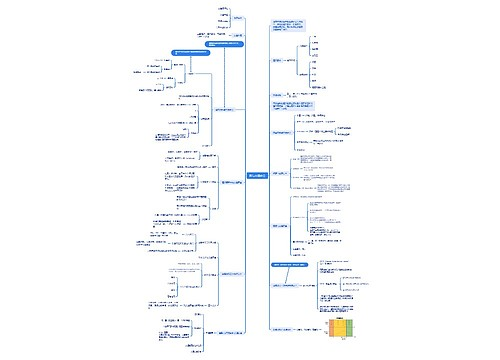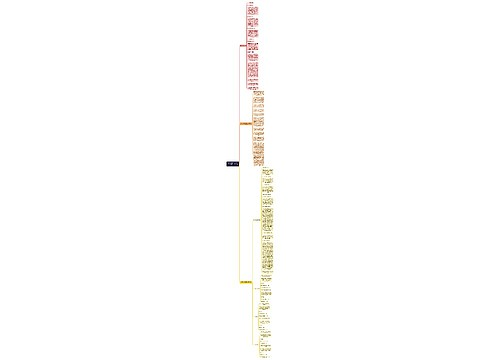### 相关思维导图专辑